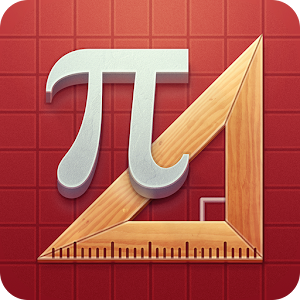## Pythagorea - Lumos Educational App Store1
Price -
\$

#### DESCRIPTION:

Study geometry while playing on squared paper. > 330+ tasks: from very simple to really geometric puzzles > 25 subjects to explore > 76 geometric terms in a glossary > Easy to use > Friendly...

#### OVERVIEW:

Pythagorea is a free educational mobile app By .It helps students in grades 8 practice the following standards 8.G.B.6,8.G.B.7,8.G.B.8.

This page not only allows students and teachers download Pythagorea but also find engaging Sample Questions, Videos, Pins, Worksheets, Books related to the following topics.

1. 8.G.B.6 : Explain a proof of the Pythagorean Theorem and its converse. .

2. 8.G.B.7 : Apply the Pythagorean Theorem to determine unknown side lengths in right triangles in real-world and mathematical problems in two and three dimensions..

3. 8.G.B.8 : Apply the Pythagorean Theorem to find the distance between two points in a coordinate system..

8

#### STANDARDS:

8.G.B.6
8.G.B.7
8.G.B.8
8.G.A.1.B
8.G.A.1.C
8.G.A.2
8.G.A.3
8.G.A.4
8.G.A.5

Developer:

Software Version:

Category:

### RELATED APPSEdSearch WebSearch